Ascending Order - Definition with Examples

The Complete K-5 Math Learning Program Built for Your Child

• 30 Million Kids

Loved by kids and parent worldwide

• 50,000 Schools

Trusted by teachers across schools

• Comprehensive Curriculum

Aligned to Common Core

What is Ascending Order?

Ascending order means to arrange numbers in increasing order, that is, from smallest to largest.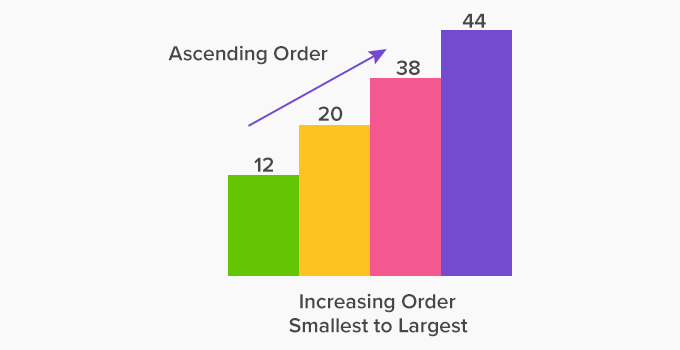To arrange numbers in any order, we first need to compare them.

First Compare, then Order

Arranging numbers in ascending order:

• Count the number of digits in each number. The number with the least number of digits is the smallest. Write it first.  Continue this till all the numbers left for comparison have the same number of digits.

• For the numbers having the same number of digits, start with comparing the numbers from the leftmost digit. Write the number with the smallest digit.

• If the leftmost digits are same, move to the digits to the right and compare them. Write the number with smaller digit.

• Continue doing this with the remaining numbers till we arrange all the numbers.

Example: Arrange 22554, 231, 22, 245, 22354 in ascending order.

The number 22 has the least number of digits. So, write it first as it is the smallest number.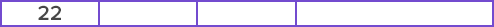Next, 231 and 245 both are 3-digit numbers. They both have 2 at hundreds place. So, we move to the digit to the right. Compare 231 and 245                  Since, 3 < 4;  So, 231 < 245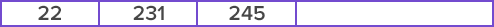Next, compare 22554 and 22354 as both have 5 digits. On comparing, 22554 and 22354, we find 3 < 5

So, 22354 < 22554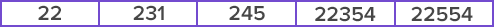We can then also arrange the numbers on the number line as shown: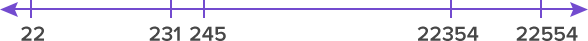Ordering fractions in ascending order

• Ordering fractions with same denominators

For the fractions having the same denominator, the fraction with the smallest numerator is the smallest.

Example: Arrange 325, 1⁄7 in ascending order.

Comparing the numerators, we get 1 < 2 < 3 < 5

Therefore, 1⁄7 < 27 < 37 < 57

• Ordering fractions with same numerators

When the fractions have the same numerator, the fraction with the highest denominator is the smallest.

Example: Arrange 33334 in ascending order.

Here, the numerator is 3 in all the fractions. So, we compare the denominator.

On comparing the denominators, we get: 4 < 5 < 7 < 8

Therefore, 3335 < 34

• Ordering fractions with different numerators and denominators

Convert the fractions to the like denominators (or numerators) and then compare and order them.

Example: Arrange 254635 and 13 in ascending order.

Here, the denominators are 5, 6 and 3.

LCM of 3, 5 and 6 is 30.

So, we find the equivalent fractions.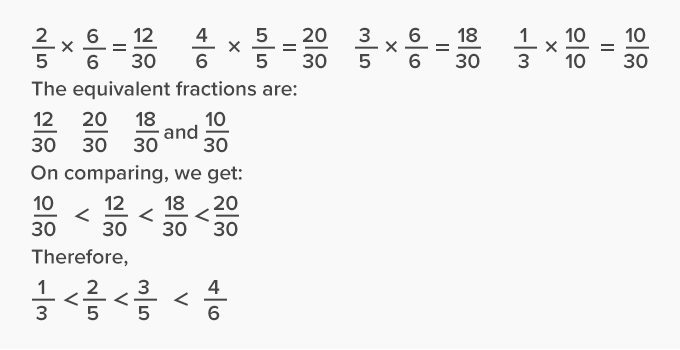Ordering decimals in ascending order

• First compare the whole number part in each decimal. The number with smallest whole number is the smallest.

• If the whole number parts are the same, compare the decimal parts just as we compare the whole numbers.

Example: Arrange 22.44, 22.04, 22.40, and 2.45 in ascending order.

First compare whole numbers:

22.44, 22.04, 22.40, and 2.45

2 is the smallest, we write it first.

 2.45

22.44, 22.04, 22.40 all have 22.

So, compare the decimal parts.

0.04 < 0.40 < 0.44

Therefore, 22.04 < 22.40 < 22.44

The ascending order is:

 2.45 22.04 22.4 22.44

 Fun Facts To remember ascending order, remember the flight of an “airplane”, from low to high.

Won Numerous Awards & Honors